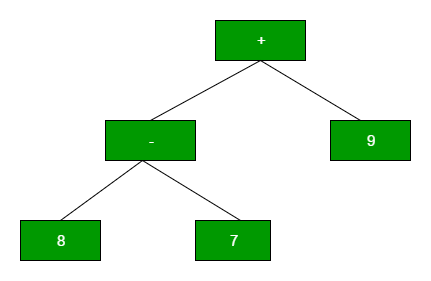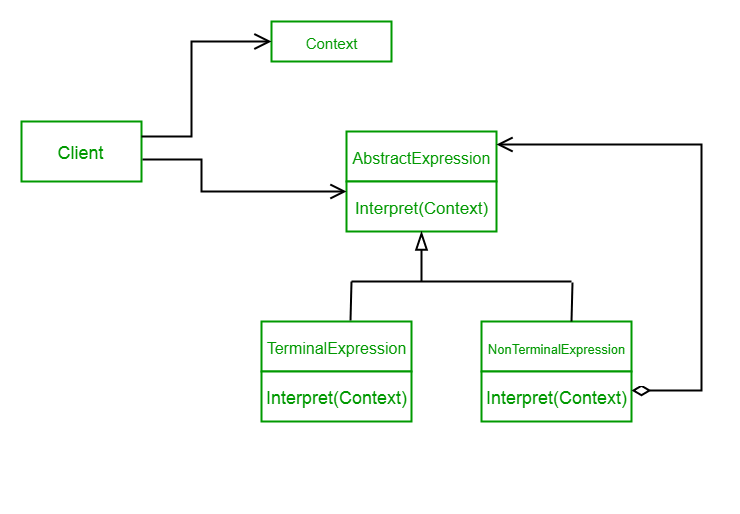Related Articles
Interpreter Design Pattern
• Last Updated : 22 Feb, 2018

Interpreter design pattern is one of the behavioral design pattern. Interpreter pattern is used to defines a grammatical representation for a language and provides an interpreter to deal with this grammar.

• This pattern involves implementing an expression interface which tells to interpret a particular context. This pattern is used in SQL parsing, symbol processing engine etc.
• This pattern performs upon a hierarchy of expressions. Each expression here is a terminal or non-terminal.
• The tree structure of Interpreter design pattern is somewhat similar to that defined by the composite design pattern with terminal expressions being leaf objects and non-terminal expressions being composites.
• The tree contains the expressions to be evaluated and is usually generated by a parser. The parser itself is not a part of the interpreter pattern.

For Example :
Here is the hierarchy of expressions for “+ – 9 8 7” :Implementing the Interpreter Pattern

UML Diagram Interpreter Design PatternDesign components

• AbstractExpression (Expression): Declares an interpret() operation that all nodes (terminal and nonterminal) in the AST overrides.
• TerminalExpression (NumberExpression): Implements the interpret() operation for terminal expressions.
• NonterminalExpression (AdditionExpression, SubtractionExpression, and MultiplicationExpression): Implements the interpret() operation for all nonterminal expressions.
• Context (String): Contains information that is global to the interpreter. It is this String expression with the Postfix notation that has to be interpreted and parsed.
• Client (ExpressionParser): Builds (or is provided) the AST assembled from TerminalExpression and NonTerminalExpression. The Client invokes the interpret() operation.

Let’s see an example of Interpreter Design Pattern.

 `// Expression interface used to``// check the interpreter.``interface` `Expression``{``    ``boolean` `interpreter(String con);``}`` ` `// TerminalExpression class implementing``// the above interface. This interpreter ``// just check if the data is same as the ``// interpreter data.``class` `TerminalExpression ``implements` `Expression ``{``    ``String data;`` ` `    ``public` `TerminalExpression(String data)``    ``{``        ``this``.data = data; ``    ``}`` ` `    ``public` `boolean` `interpreter(String con) ``    ``{``        ``if``(con.contains(data))``        ``{``            ``return` `true``;``        ``}``        ``else``        ``{``            ``return` `false``;  ``        ``}``    ``}``}``// OrExpression class implementing``// the above interface. This interpreter ``// just returns the or condition of the ``// data is same as the interpreter data.``class` `OrExpression ``implements` `Expression ``{``    ``Expression expr1;``    ``Expression expr2;`` ` `    ``public` `OrExpression(Expression expr1, Expression expr2) ``    ``{``        ``this``.expr1 = expr1;``        ``this``.expr2 = expr2;``    ``}``    ``public` `boolean` `interpreter(String con) ``    ``{        ``        ``return` `expr1.interpreter(con) || expr2.interpreter(con);``    ``}``}`` ` `// AndExpression class implementing``// the above interface. This interpreter ``// just returns the And condition of the ``// data is same as the interpreter data.``class` `AndExpression ``implements` `Expression ``{``    ``Expression expr1;``    ``Expression expr2;`` ` `    ``public` `AndExpression(Expression expr1, Expression expr2) ``    ``{ ``        ``this``.expr1 = expr1;``        ``this``.expr2 = expr2;``    ``}``    ``public` `boolean` `interpreter(String con) ``    ``{        ``        ``return` `expr1.interpreter(con) && expr2.interpreter(con);``    ``}``}`` ` `// Driver class``class` `InterpreterPattern``{`` ` `    ``public` `static` `void` `main(String[] args) ``    ``{``        ``Expression person1 = ``new` `TerminalExpression(``"Kushagra"``);``        ``Expression person2 = ``new` `TerminalExpression(``"Lokesh"``);``        ``Expression isSingle = ``new` `OrExpression(person1, person2);``         ` `        ``Expression vikram = ``new` `TerminalExpression(``"Vikram"``);``        ``Expression committed = ``new` `TerminalExpression(``"Committed"``);``        ``Expression isCommitted = ``new` `AndExpression(vikram, committed);    `` ` `        ``System.out.println(isSingle.interpreter(``"Kushagra"``));``        ``System.out.println(isSingle.interpreter(``"Lokesh"``));``        ``System.out.println(isSingle.interpreter(``"Achint"``));``         ` `        ``System.out.println(isCommitted.interpreter(``"Committed, Vikram"``));``        ``System.out.println(isCommitted.interpreter(``"Single, Vikram"``));`` ` `    ``}``}`

Output:

```true
true
false
true
false
```

In the above code , We are creating an interface Expression and concrete classes implementing the Expression interface. A class TerminalExpression is defined which acts as a main interpreter and other classes OrExpression, AndExpression are used to create combinational expressions.